Diesel engine. Diesel cycle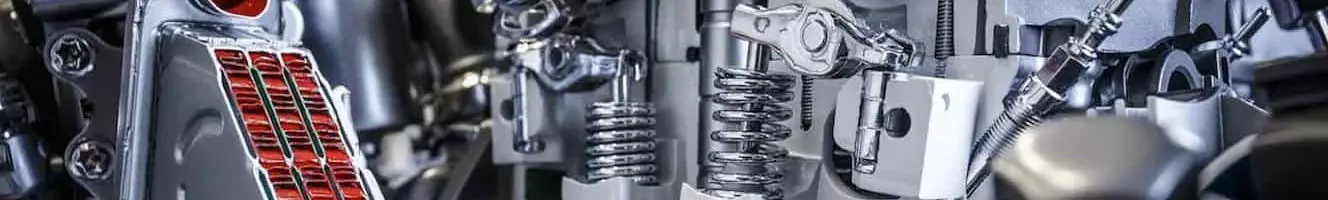Endothermic engine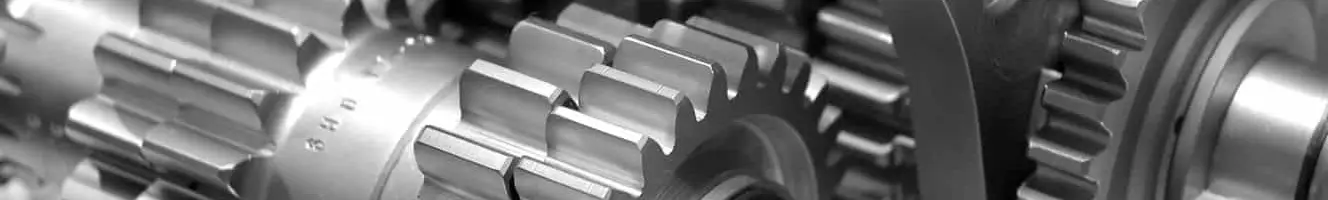Gearbox

# Theoretical

## Difference between real and theoretical Otto cycles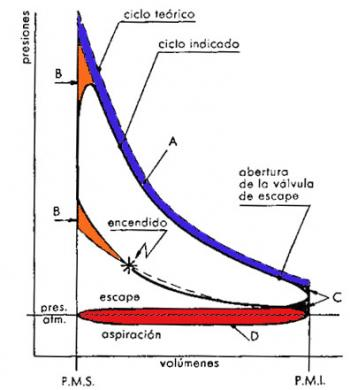Between the Otto real cycle and the corresponding theoretical Otto there are substantial differences. Some of these differences can be observed when comparing the diagram of the real cycle with the theoretical cycle diagram. The other differences that we are going to analyze refer to the differences between the temperature and pressure values of the two Otto engine cycles.

Differences in the form of the diagram

The difference in shape of the diagram between a real Otto cycle and a theoretical Otto cycle consists of a different profile in the expansion and compression curves, in…

+ info

## Theoretical diesel cycle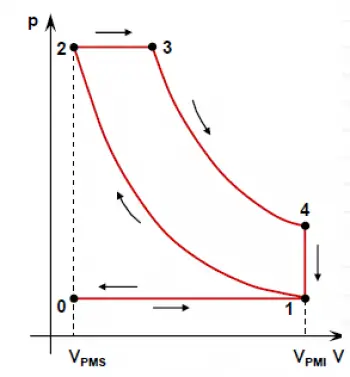The theoretical diesel cycle is the theoretical cycle of a diesel engine, also known as a compression ignition engine.

The theoretical cycle of a thermal engine is a theoretical approximation of its operation to calculate its performance.

The cycle of an internal combustion engine is constituted by the physical and chemical transformations suffered by the fuel during its passage inside the engine.

The study of a real cycle taking into account all the numerous variables, represents a very complex problem. For this reason, it is usually simplified by resorting to theoretical…

+ info

## Theoretical and real cycles Cycles

Cycles theoretical endothermic engines

For theoretical cycles, commonly used approaches aproximacióna by the real conditions are three:

• real cycle
• air cycle
• air-fuel cycle.

A theoretical these cycles are compared in practice the actual cycles, which are obtained experimentally by means of the indicators, for this reason, the actual cycle also indicated cycle is called.

Although the theoretical cycles do not correspond to actual cycles, provide a useful reference for the thermodynamic study of internal combustion engines,…

+ info

## Otto cycle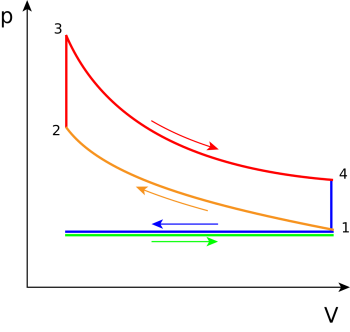The theoretical Otto cycle is the ideal cycle of the Otto engine. The Otto engine is also known as a spark ignition engine because the ignition of the fuel is done through a spark caused by a spark plug. It is also known as a gasoline engine because of the type of fuel it uses.

One way to study the performance of this engine is by analyzing its theoretical cycle. The theoretical cycle is an approximation to the real cycle with many simplifications. In practice, there are so many variables that affect the performance of the engine that calculating the actual cycle is practically impossible.…

+ info

## Differences between the real and theoretical diesel cycle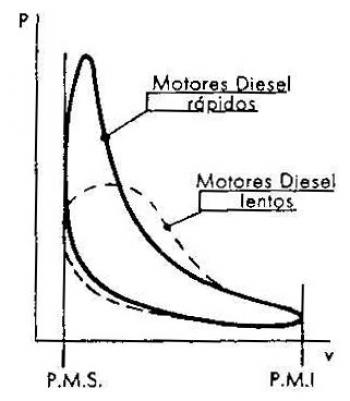Between real and theoretical diesel cycles there are differences and similarities in the form and in the values of pressures and temperatures. Differences that also appear in the otto cycle. Some of these similarities correspond to those of the otto cycle; for example, the similarities due to the variation of the specific heats, to the loss of heat and to the opening time of the exhaust valve.

Others differ in part and are caused by dissociation and loss by pumping. Finally, one is peculiar to the diesel engine, namely; the one concerning that the combustion is not verified at constant…

+ info

## Cycles of the thermal engines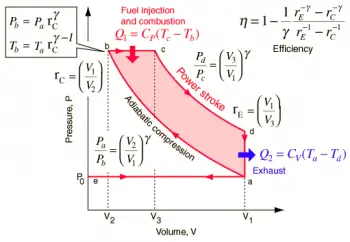The operating system of the thermal engines is based on a cycle that is constantly repeated. In the case of internal combustion engines this cycle consists of the fuel input (admission), combustion of the fuel, expansion of the cylinder and expulsion of the gases produced. This cycle generates a mechanical movement, if the cycle repeats we will have a constant mechanical movement.

A thermal engine can work through many different cycles. In this section we will explain the different operating cycles that an internal combustion engine can have, the real cycles and the theoretical cycles.

+ info

## Sabathé mixed cycle

The actual operating conditions of diesel engines differ markedly from those that are represented in the ideal Otto and Diesel cycles. For diesel engines, the combustion process transformational approaches a constant pressure only in case of unusually large motors and slow.

The actual plot shows that, under normal conditions, the combustion takes place, in Diesel engines, according to a process that approximates a transformational combustion and a constant volume at pressures n…

+ info

## Comparison between the three theoretical cycles

To compare cycle just discussed, it is necessary to refer some of the factors whose value depends on the shape and surface, such as: the compression ratio, the maximum pressure, the amount of heat supplied and the heat subtracted useful work.

The following figure are plotted the curves of the ideal thermal performance variations by varying the ratio of co

RINTING for three cycles: Otto, Diesel and Sabathé. For Diesel cycles and Sabathé has chosen a constant pressure…

+ info

## Real cycles of thermal engines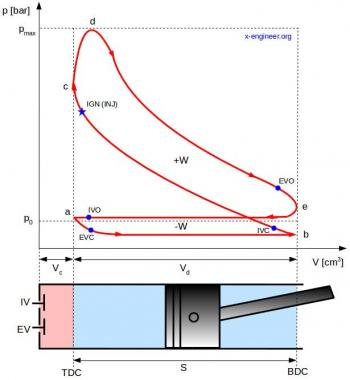The actual cycle of a thermal engine is the one that reflects the effective conditions of its operation. These conditions are identified with the diagram of average pressures in the cylinder in correspondence to the different positions of the piston. The pressure shown in a mean pressure diagram is the so-called average pressure indicated.

The indicated diagram is the graph that represents this cycle.

Simplified operation of the indicator

The indicator is a device used to obtain the indicated diagram of a thermal engine cycle.

In the indicator of the indicated diagram,…

+ info